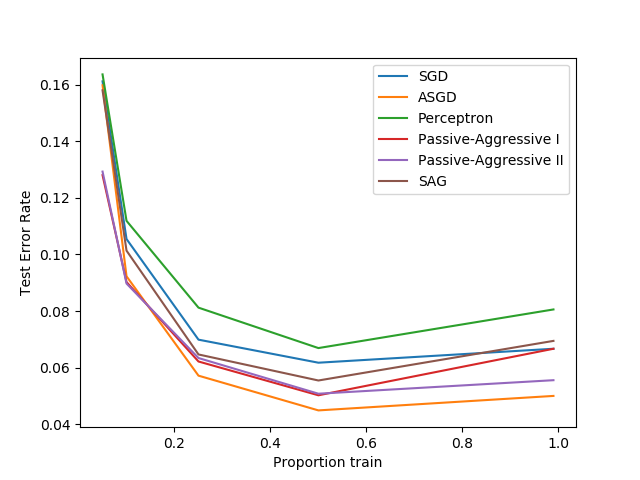# Comparing various online solvers¶

An example showing how different online solvers perform on the hand-written digits dataset.Out:

training SGD
training ASGD
training Perceptron
training Passive-Aggressive I
training Passive-Aggressive II
training SAG


# Author: Rob Zinkov <rob at zinkov dot com>

import numpy as np
import matplotlib.pyplot as plt
from sklearn import datasets

from sklearn.model_selection import train_test_split
from sklearn.linear_model import SGDClassifier, Perceptron
from sklearn.linear_model import PassiveAggressiveClassifier
from sklearn.linear_model import LogisticRegression

heldout = [0.95, 0.90, 0.75, 0.50, 0.01]
rounds = 20

classifiers = [
("SGD", SGDClassifier(max_iter=100)),
("ASGD", SGDClassifier(average=True)),
("Perceptron", Perceptron()),
("Passive-Aggressive I", PassiveAggressiveClassifier(loss='hinge',
C=1.0, tol=1e-4)),
("Passive-Aggressive II", PassiveAggressiveClassifier(loss='squared_hinge',
C=1.0, tol=1e-4)),
("SAG", LogisticRegression(solver='sag', tol=1e-1, C=1.e4 / X.shape))
]

xx = 1. - np.array(heldout)

for name, clf in classifiers:
print("training %s" % name)
rng = np.random.RandomState(42)
yy = []
for i in heldout:
yy_ = []
for r in range(rounds):
X_train, X_test, y_train, y_test = \
train_test_split(X, y, test_size=i, random_state=rng)
clf.fit(X_train, y_train)
y_pred = clf.predict(X_test)
yy_.append(1 - np.mean(y_pred == y_test))
yy.append(np.mean(yy_))
plt.plot(xx, yy, label=name)

plt.legend(loc="upper right")
plt.xlabel("Proportion train")
plt.ylabel("Test Error Rate")
plt.show()


Total running time of the script: ( 0 minutes 17.905 seconds)

Estimated memory usage: 8 MB

Gallery generated by Sphinx-Gallery